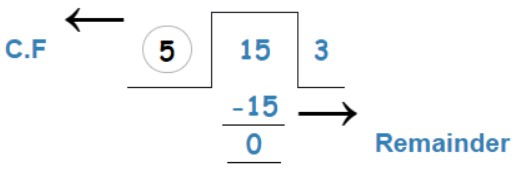# GCF Calculator

Enter numbers, select the method and hit the Calculate button to find the GCF using GCF calculator.

Min required 2 values comma separated

Give Us Feedback

GCF (HCF) calculator in an online tool that finds the greatest common factor in the given numbers. It uses three methods to calculate the GCF.

1. List of Factors
2. Division Step
3. Prime Factorization

## What is GCF?

GCF is short form of greatest common factor. It is the largest positive integer that divides all of the given integers. It is also known as greatest common divisor (GCD) or highest common factor (HCF).

## How to calculate GCF/GCD/HCF?

To calculate greatest common factor, follow the example below or use our greatest common divisor calculator above.

Example:

Find the greatest common divisor among 5, 10, and 15.

Solution:

### List of Factors

Step 1: Write down factors of all numbers.

5 = 1, 5

10 = 1, 2, 5, 10

15 = 1, 3, 5, 15

Step 2: Highlight all common factors and choose the greatest one.

5 = 1, 5

10 = 1, 2, 5, 10

15 = 1, 3, 5, 15

Here, 5 is the only common factor in all of the factors. So,

GCF (5, 10, 15) = 5

### Prime Factorization

Step 1: List all prime factors of given numbers.

5 = 1 × 5

10 = 2 × 5

15 = 3 × 5

Step 2: Highlight common numbers. If there are several common numbers in prime factors of all numbers, multiply them.

5 = 1 × 5

10 = 2 × 5

15 = 3 × 5

GCF (5, 10, 15) = 5

### Division Step Method

Step 1: Divide the largest number with the smallest number.5 is the common factor (C.F) of 5 and 15.

Step 2: Now divide the remaining number 10 with the C.F 5.The divisor that completely divides the number in the end will be GCF.

GCF (5, 10, 15) = 5

## GCF Table

 GCF of 24 and 36 12 GCF of 21 and 100 1 GCF of 18 and 24 6 GCF of 18 and 36 18 GCF of 12 and 18 6 GCF of 12 and 16 4 GCF of 12 and 20 4 GCF of 15 and 20 5 GCF of 16 and 24 8 GCF of 9 and 15 3 GCF of 27 and 36 9 GCF of 8 and 10 2 GCF of 8 and 12 4 GCF of 8 and 14 2 GCF of 3,6 and 9 3 HCF of 36 and 48 12 GCF of 2 and 3 1

### Other Languages